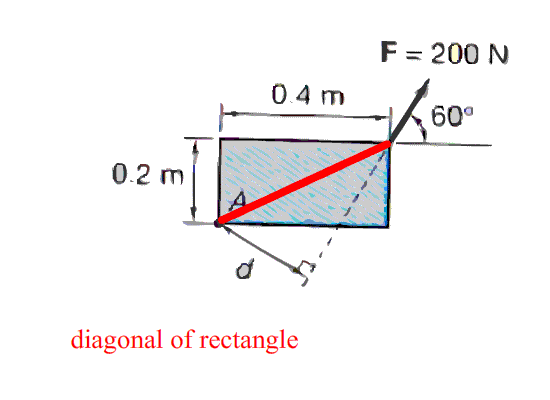If given a horizontal length between a point on a particle and another point with a given moment force and its angle, you can take the sine of 45 degrees of the horizontal length to find the diagonal of the rectangle. The theory that the diagonal multiplied by the sine of the angle of the moment force, allows us to solve the length of the line of action from the force to the moment arm. Thus, allowing us to easily solve the moment arm which is the force of the moment.